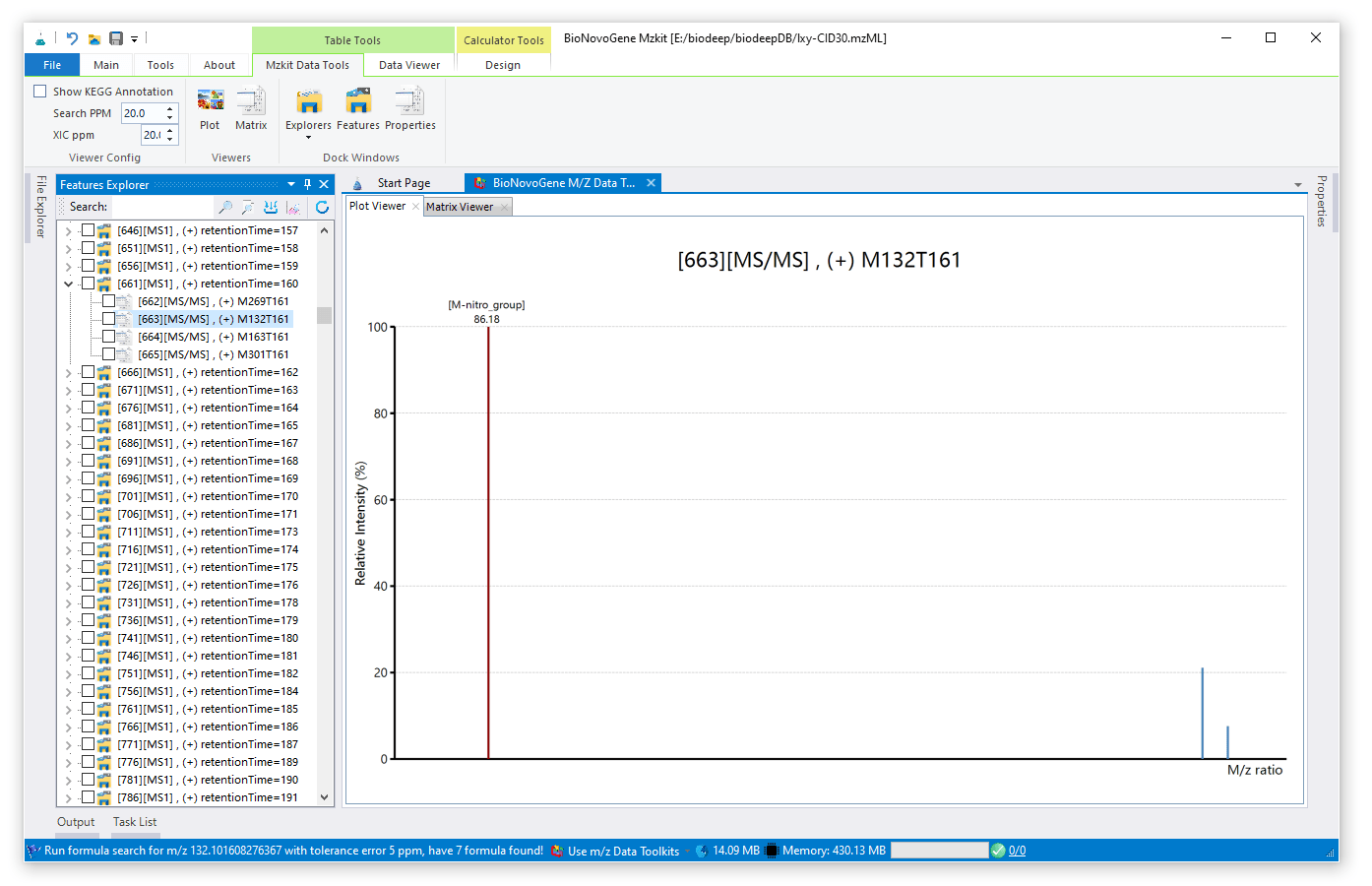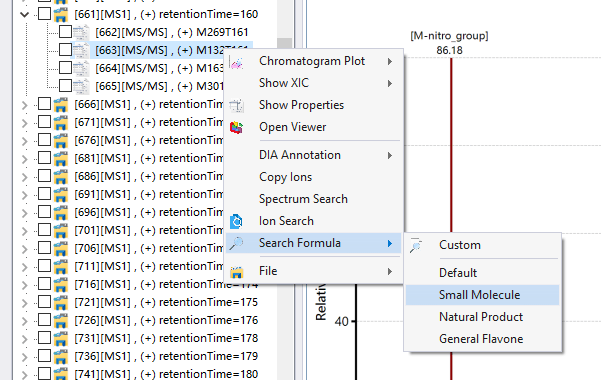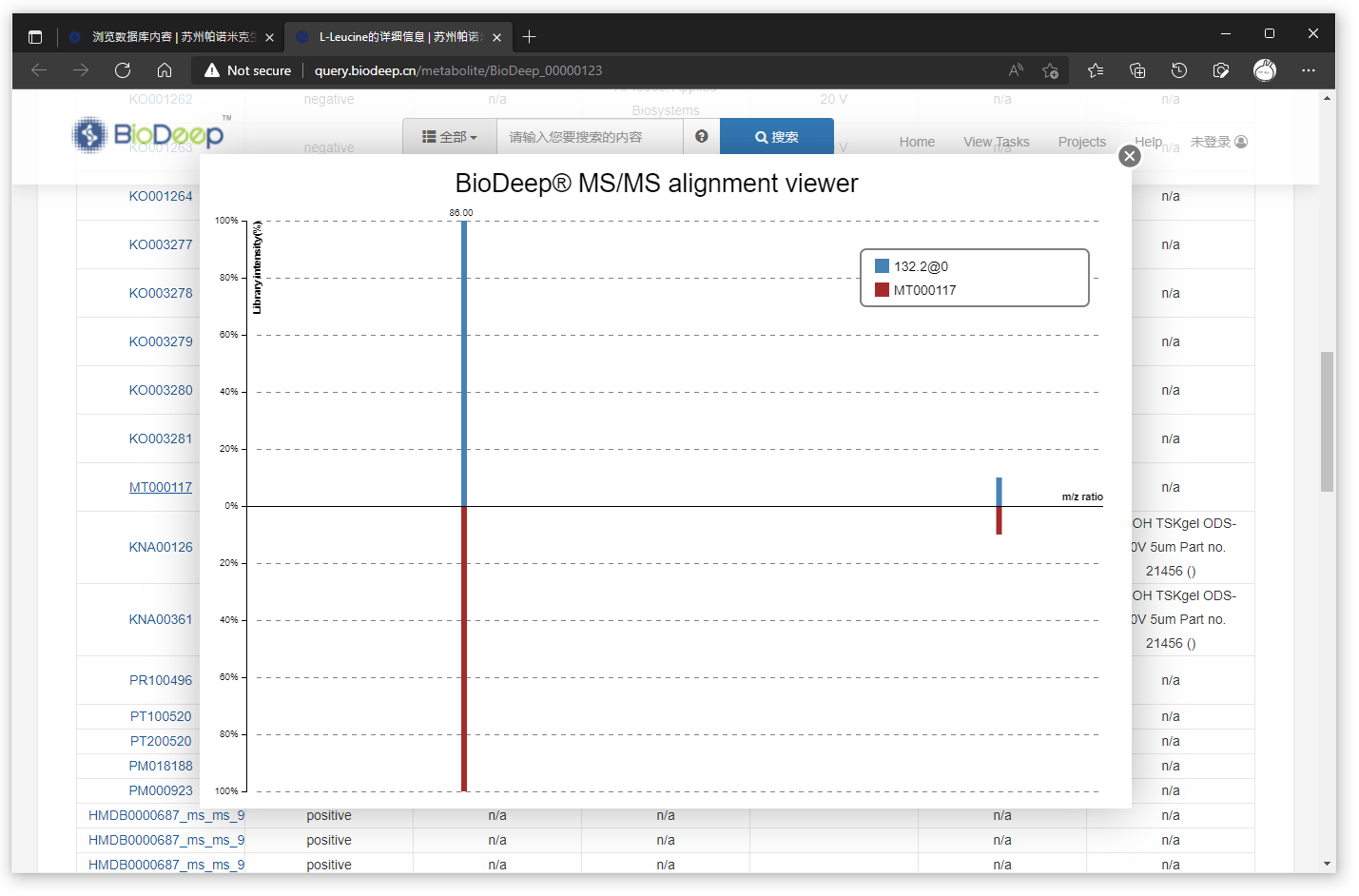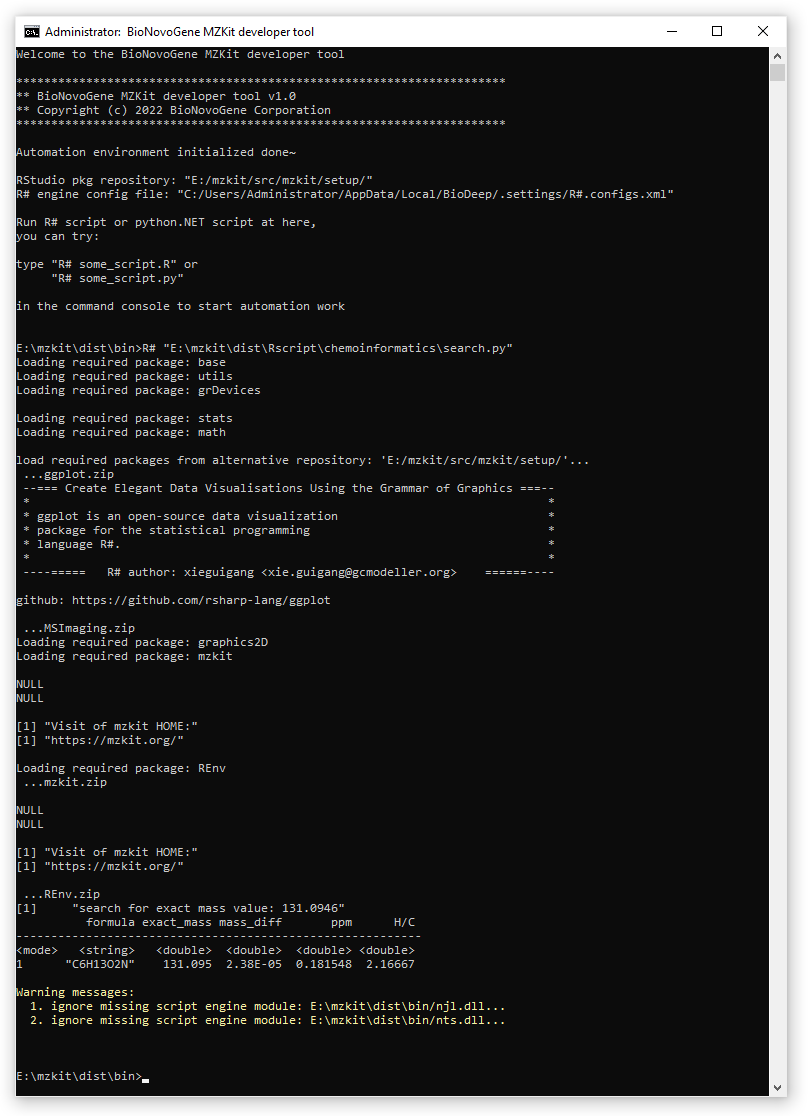## 原理基础

### 离子精确分子质量### 通过m/z逆推代谢物化学式

• 基于mz值的正向的计算公式 (exact_mass + adduct) / charge = mz
• 可以逆推为 exact_mass = mz * charge - adduct

## 在MZKit中基于mz进行formula搜索Formula 根据m/z或者exact mass搜索得到的化学式列表，对应的链接会链接查询BioDeep在线数据库
Exact Mass 对应的化学式所计算出的精确分子质量
PPM mz所计算出的精确分子质量与化学式计算出的离子精确分子质量之间的误差
Charge 离子的电荷数量
M 本体化合物的数量
Precursor Type 对应的离子加合物形式的符号简记http://query.biodeep.cn/search?expression=[formula]&category=metabolite&formula=C6H13NO2## 基于MZKit的化学信息学工具箱进行批量搜索

### 导入MZKit代码库

import mzkit
from mzkit import formula

### 调用MZKit化学信息学代码库

exact_mass = formula::eval("C40H78NO8P")

formulas = formula::candidates(exact_mass, ppm = 5, C = [6,72], H = [3,120], O = [1,20], N = [0, 18], P = [0, 18], S = [0, 20])
list = as.data.frame(formulas)
list = list[ order(list[, "ppm"]) , ]

print(search for exact mass value: ${exact_mass}) print(list) 上面的代码就可以让我们实现一个搜索某一个exact mass值在5ppm的误差下的所有候选formula，并且搜索结果按照ppm误差升序排序的功能。 formula::candidates函数之中，除了输入必要的exact mass参数和ppm误差之外，我们还可以自己通过列表参数设定对应的化学元素的数量的搜索范围。 ### 脚本运行示例 下面给出了具体的完整代码用于从exact mass搜索出所有的候选化学式结果信息： import mzkit from mzkit import formula exact_mass = 131.0946 formulas = formula::candidates(exact_mass, ppm = 10, C = [6,72], H = [3,120], O = [1,20], N = [0, 18], P = [0, 18], S = [0, 20]) list = as.data.frame(formulas) list = list[ order(list[, "ppm"]) , ] print(search for exact mass value:${exact_mass})
print(list)Latest posts by 谢桂纲 (see all)

### Order by Date Name Attachments

#### 3 Responses

1.lina says:

whoah this blog is wonderful i really like reading your articles. Keep up the great paintings! You realize, a lot of people are hunting round for this info, you could help them greatly.

来自德国
2.lina says:

Great selection of modern and classic books waiting to be discovered. All free and available in most ereader formats. download free books https://www.skylineuniversity.ac.ae/advisory-council

来自德国
3.lina says:

Great selection of modern and classic books waiting to be discovered. All free and available in most ereader formats. download free books https://www.philadelphia.edu.jo/library/directors-message-library

来自德国